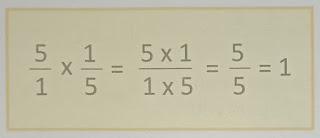# Lesson Planning of Multiplication of Fraction

Lesson Planning of Multiplication of Fraction with Whole Number

Subject Mathematics

Students` Learning Outcomes

• Multiply fractions with whole numbers.

Information for Teachers

• Multiplying a fraction by whole number follows the same rules as multiplying two fractions.• A whole number can be considered as a fraction with a denominator of 1.
• Therefore when a fraction is multiplied by a whole number the numerator of the fraction is multiplied by the number.
• The denominator is multiplied by 1 which does n`t change the denominator.
• While teaching the lesson, teacher should also consult with textbook at all steps where and when required.

Material/ Resources

Writing board, chalk, marker, duster, chart paper, textbook

Introduction

• Write on the board: 1 whole and ask:
1. How many halves (1/2) it has? 
2. Can I say it is as 2 x ½? [Yes]
• Write the following on the board and make students repeat with you.
• Ask the students; what we get if we divide any number with 1. i.e. 7 ÷ 1 = 7 / 1 = 7 (Repeat with other numbers)
• Announce that today we will talk about multiplication of fractions with whole numbers.

Development

 Activity 1 Through following points recap the students` previous knowledge; A fraction is a part of a whole, as; if you had an apple and you cut into 5 equal parts, each pieces would represent 1 / 5. (Notice that if you put the five pieces back together or 1 / 5 + 1 / 5 + 1 / 5 + 1/5 + 14 / 5, then you would get 5 / 5 of an apple, which is 1 apple.) If you want to show 3 / 5 of a circle, into how many equal parts would you have to divide that circle? How many of these parts would you need to shade? Ask the students to recall the apple example above. If we add 1 / 5 + 1 / 5 + 1 / 5 + 1 / 5 + 1 / 5, isn`t that the same as saying 5 x (1 / 5)? [yes] Tell the students that; to multiply 5 and 1 / 5, you may need to rewrite 5 as 5 / 1. Multiply the numerators to find the new denominator, which turns out to be 5 / 5. Write on the board:Solve another example: 2 / 3 x 12 on the board.Solve few more examples on board, of different whole numbers and fractions, with the help of the students.

 Activity 2 Ask the students some questions, and show working on the board. There are 10 sweets in a bag. Zane eats half of them. How many sweets are left? There are 8 children on the playground. Half of them are called in to lunch. How many are left on the playground? There are 120 pages in Sara’s book. She had read 1 / 3 of it. How many pages does she have left to read? After solving and discussing these questions assign questions from the textbook for individual work. Let them share the answers with the whole class and help them if they need.

Sum up / Conclusion

• Conclude lesson with the following points.
• Multiplying a fraction by whole number follows the same rules as multiplying two fractions.
• Each whole number can be written in fraction form, i.e. 12 = 12/1, 35 = 35 / 1
• Therefore when a fraction is multiplied by a whole number the numerator of the fraction is multiplied by the number.

Assessment

• Give the following questions to solve by showing all steps of multiplication.